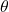Question

6) A plane taking off leaves the runway at 329 to the horizontal, travelling at
180kmh 1. How long will it take to climb to an altitude of 1000m?​

1.## 2.7secs

Explanation:

The question is not properly written. Here is the correct question

A plane taking off leaves the runway at 32° to the horizontal, travelling at

180km/h. How long will it take to climb to an altitude of 1000m?​

This motion of the plane is a projectile motion. From the question, we are to calculate the time taken by the plane to reach the maximum height.

Using the formula tmax = Usin/gis the angle of projection = 32°

U is the velocity of the plane (in m/s) = 180* 1000/3600 = 50m/s

g is the acceleration due to gravity = 9.8m/s²

tmax = 50sin32°/9.8

tmax = 26.496/9.8

tmax = 2.7sec